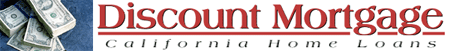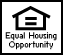Sunday, October 17, 2021 | Login
 Search

## Mortgage Calculations by Hand

First you must define some variables to make it easier to set up: P = principal, the initial amount of the loan I = the annual interest rate (from 1 to 100%) L = length, the length (in years) of the loan, or at least the length over which the loan is amortized.

The following assumes a typical conventional loan where the interest is compounded monthly. First we'll define two more variables to make the calculations easier: J = monthly interest in decimal form = I / (12 x 100) N = number of months over which loan is amortized = L x 12

Now for the big monthly payment (M) formula ... it is:

J M = P x ------------------------ 1 - ( 1 + J ) ^ -N where 1 is the number one (it does not appear too clearly on some browsers)

So to calculate it, you would first calculate 1 + J then take that to the -N (minus N) power, subtract that from the number 1. Now take the inverse of that (if you have a 1/X button on your calculator push that). Then multiply the result times J and then times P.

The one-liner for a program would be (adjust for your favorite language):

M = P * ( J / (1 - (1 + J) ** -N))

So now you should be able to calculate the monthly payment, M. To calculate the amortization table you need to do some iterations (i.e. a simple loop). Here are the simple steps :

Step 1: Calculate H = P x J, this is your current monthly interest
Step 2: Calculate C = M - H, this is your monthly payment minus your monthly interest, so it is the amount of principal you pay for that month
Step 3: Calculate Q = P - C, this is the new balance of your principal of your loan.
Step 4: Set P equal to Q and go back to Step 1: You thusly loop around until the value Q (and hence P) goes to zero.

Many people have asked how to find N (number of payments) given the payment, interest and loan amount. The answer to the actual formula is in the book: The Vest Pocket Real Estate Advisor by Martin Miles (Prentice Hall). Here's the formula:

N = -1/Q * (LN(1-(B/M)*(R/Q)))/LN(1+(R/Q))

Where:

• Q = amount of annual payment periods
• R = interest rate
• B = principle
• M = payment amount
• N = amount payment period
• LN = natural logarithm
 Discount Mortgage Office (888)360-3337 | Cell (408)316-5454 Real Estate Broker, California Department of Real Estate 9162270931 Bkr. Lic #01098186 [SIC code=6162 DUN #93-794-6796] NMLS# 314249Discount Mortgage Office (888)360-3337 | Cell (408)316-5454 Real Estate Broker, California Department of Real Estate 9162270931 Bkr. Lic #01098186 [SIC code=6162 DUN #93-794-6796] NMLS# 314249